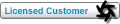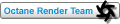## matrix 4x4 to matrix 4x3 conversion Tweet

Forum for OctaneRender Lua scripting examples, discussion and support.

### matrix 4x4 to matrix 4x3 conversion

How can I convert a matrix 4x4 (from Maya) to octane's 4x3 (scatter)?
Is it a matter of rotating it and stripping a row or is this somewhat more complicated ?

thank you!
OS: Win7 x64, RAM: 8Gb, GPU: GTX 460 2048 Mb
ruthenoidPosts: 21
Joined: Sat Apr 17, 2010 6:51 am
Location: cologne, ger

### Re: matrix 4x4 to matrix 4x3 conversion

here is my simple mel script to convert maya transform matrix to octane matrix, you can see how it is re-ordered

Code: Select all
`{string \$selection[] = `ls -sl -tr`;int \$n = 0;string \$octMatrix[] ; for (\$transform in \$selection) {    float \$matrix[] = `xform -q -ws -m \$transform`;    \$octMatrix[\$n] = \$matrix+" "+\$matrix+" "+\$matrix+" "+\$matrix+" "+\$matrix+" "+\$matrix+" "+\$matrix+" "+\$matrix+" "+\$matrix+" "+\$matrix+" "+\$matrix+" "+\$matrix+" ";    \$n=\$n+1;    } print \$octMatrix;}`
Pascal ANDRE
--------------------
Maya 2019, Windows 7, 128 GB RAM
4x GTX 1070, driver 430.39
--------------------

--------------------
Join MAYA OCTANE USERS Skype discussion here :
https://join.skype.com/LXEQaqqfN15w
--------------------
calusPosts: 1293
Joined: Sat May 22, 2010 9:31 am
Location: Paris

### Re: matrix 4x4 to matrix 4x3 conversion

Awsome!
Thank you, calus!
OS: Win7 x64, RAM: 8Gb, GPU: GTX 460 2048 Mb
ruthenoidPosts: 21
Joined: Sat Apr 17, 2010 6:51 am
Location: cologne, ger

### Re: matrix 4x4 to matrix 4x3 conversion

Yes normally this is the case. Octane only supports affine transforms, it does not support projective transform matrices. In mathematical terms this means the last row of the matrix will be (0, 0, 0, 1). This row is not explicitly stored in Octane.roeland
OctaneRender TeamPosts: 1738
Joined: Wed Mar 09, 2011 10:09 pm